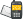It is currently 24 May 2020, 18:14### GMAT Club Daily Prep

#### Thank you for using the timer - this advanced tool can estimate your performance and suggest more practice questions. We have subscribed you to Daily Prep Questions via email.

Customized
for You

we will pick new questions that match your level based on your Timer History

Track

every week, we’ll send you an estimated GMAT score based on your performance

Practice
Pays

we will pick new questions that match your level based on your Timer History

#### Not interested in getting valuable practice questions and articles delivered to your email? No problem, unsubscribe here.# If a triangle in the xy-coordinate system has vertices atQuestion banks Downloads My Bookmarks Reviews Important topics
Author Message
TAGS:
FounderJoined: 18 Apr 2015
Posts: 11096
Followers: 237

Kudos [?]: 2769 , given: 10521

If a triangle in the xy-coordinate system has vertices at [#permalink]
Expert's post00:00

Question Stats:84% (01:08) correct15% (01:30) wrongbased on 26 sessions
If a triangle in the xy-coordinate system has vertices at (– 2, –3), (4, –3) and (28, 7), what is the area of the triangle?

A. 30

B. 36

C. 48

D. 60

E. 65
[Reveal] Spoiler: OA

_________________

Need Practice? 20 Free GRE Quant Tests available for free with 20 Kudos
GRE Prep Club Members of the Month: Each member of the month will get three months free access of GRE Prep Club tests.InternJoined: 30 Nov 2018
Posts: 12
GRE 1: Q164 V164Followers: 1

Kudos [?]: 14  , given: 12

Re: If a triangle in the xy-coordinate system has vertices at [#permalink]
1
KUDOS
Wait this isn't multiple choice with select all that apply..
FounderJoined: 18 Apr 2015
Posts: 11096
Followers: 237

Kudos [?]: 2769 , given: 10521

Re: If a triangle in the xy-coordinate system has vertices at [#permalink]
Expert's post
Moved, thanks Sir.

Regards
_________________

Need Practice? 20 Free GRE Quant Tests available for free with 20 Kudos
GRE Prep Club Members of the Month: Each member of the month will get three months free access of GRE Prep Club tests.

InternJoined: 02 Dec 2018
Posts: 16
Followers: 0

Kudos [?]: 3 , given: 0

Re: If a triangle in the xy-coordinate system has vertices at [#permalink]
High 10 base 30 ?

Posted from my mobile deviceDirectorJoined: 09 Nov 2018
Posts: 506
Followers: 0

Kudos [?]: 54 , given: 1

Re: If a triangle in the xy-coordinate system has vertices at [#permalink]
tagedwedw wrote:
High 10 base 30 ?

Posted from my mobile deviceNo, Height 10
Base 6

Area = $$\frac{1}{2}*6*10=30$$

Last edited by AE on 08 Jan 2019, 16:56, edited 1 time in total.
Supreme Moderator
Joined: 01 Nov 2017
Posts: 371
Followers: 10

Kudos [?]: 159 , given: 4

Re: If a triangle in the xy-coordinate system has vertices at [#permalink]
Expert's post
Carcass wrote:
If a triangle in the xy-coordinate system has vertices at (– 2, –3), (4, –3) and (28, 7), what is the area of the triangle?

A. 30

B. 36

C. 48

D. 60

E. 65

(-2,-3) and (4,-3) is a straight line parallel to x axis at y=-3. Therefore let's take this as the base..so Base = 4-(-2)=4+2=6..

Now the third vertice is (28,7)..
If we are taking base as parallel to x-axis , the height will be parallel to y-axis.
Therefore height is 7-(-3)=7+3=10

Area will be $$\frac{1}{2}*6*10=30$$

A
_________________

Some useful Theory.
1. Arithmetic and Geometric progressions : https://greprepclub.com/forum/progressions-arithmetic-geometric-and-harmonic-11574.html#p27048
2. Effect of Arithmetic Operations on fraction : https://greprepclub.com/forum/effects-of-arithmetic-operations-on-fractions-11573.html?sid=d570445335a783891cd4d48a17db9825
3. Remainders : https://greprepclub.com/forum/remainders-what-you-should-know-11524.html
4. Number properties : https://greprepclub.com/forum/number-property-all-you-require-11518.html
5. Absolute Modulus and Inequalities : https://greprepclub.com/forum/absolute-modulus-a-better-understanding-11281.html

DirectorJoined: 09 Nov 2018
Posts: 506
Followers: 0

Kudos [?]: 54 , given: 1

Re: If a triangle in the xy-coordinate system has vertices at [#permalink]
chetan2u wrote:

(-2,-3) and (4,-3) is a straight line parallel to y axis at y=-3. Therefore let's take this as the base..so Base = 4-(-2)=4+2=6..

Is it parallel to y axis or x axis? Please make it clear.
Supreme Moderator
Joined: 01 Nov 2017
Posts: 371
Followers: 10

Kudos [?]: 159 , given: 4

Re: If a triangle in the xy-coordinate system has vertices at [#permalink]
Expert's post
AE wrote:
chetan2u wrote:

(-2,-3) and (4,-3) is a straight line parallel to y axis at y=-3. Therefore let's take this as the base..so Base = 4-(-2)=4+2=6..

Is it parallel to y axis or x axis? Please make it clear.

y=-3 will be parallel to z-axis.
Thanks edited
_________________

Some useful Theory.
1. Arithmetic and Geometric progressions : https://greprepclub.com/forum/progressions-arithmetic-geometric-and-harmonic-11574.html#p27048
2. Effect of Arithmetic Operations on fraction : https://greprepclub.com/forum/effects-of-arithmetic-operations-on-fractions-11573.html?sid=d570445335a783891cd4d48a17db9825
3. Remainders : https://greprepclub.com/forum/remainders-what-you-should-know-11524.html
4. Number properties : https://greprepclub.com/forum/number-property-all-you-require-11518.html
5. Absolute Modulus and Inequalities : https://greprepclub.com/forum/absolute-modulus-a-better-understanding-11281.htmlRe: If a triangle in the xy-coordinate system has vertices at   [#permalink] 08 Jan 2019, 18:50
Display posts from previous: Sort by

# If a triangle in the xy-coordinate system has vertices atQuestion banks Downloads My Bookmarks Reviews Important topicsPowered by phpBB © phpBB Group Kindly note that the GRE® test is a registered trademark of the Educational Testing Service®, and this site has neither been reviewed nor endorsed by ETS®.